# Solving the Sound of a Guitar String

## Jason Pelc December 10, 2007

### (Submitted as coursework for Physics 210, Stanford University, Fall 2007)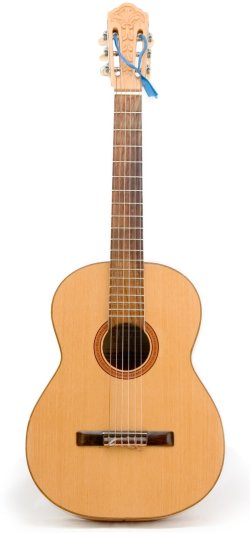Fig. 1: Photograph of a standard acoustic guitar. 

## Introduction

In this work we construct a physical model of the motion of a guitar string, and analytically recreate, from first principles, the sound produced by the string. We begin with an idealized model of a string, and proceed to a model including string stiffness which results in inharmonicity which, to a listener's ear, is generally characterized by a decrease in sound quality.

## Ideal String

We begin with an idealized physical model of a string in which the string has no dissipation, and in which the amplitude variations are small. In this limit, we can easily derive the equation of motion for the string, in which the amplitude y is expressed as a function of the position x along the string and the time t: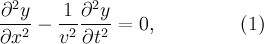where the wave velocity v=(T/μ)1/2 with T being the tension of the string and μ being the linear mass density. We seek to find the normal modes of the string, and solve the equation under the conditions that the string extends from x=0 and to x=L. A general solution to the equation is found by superimposing the normal modes subject to the boundary conditions. We write the general solution as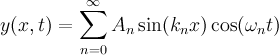where the An are determined by the initial and boundary conditions, and the angular frequency of the wave &omegan=vkn. Because the ends of the string are held fixed, y(0,t)=y(L,t)=0, we find that kn=n&pi/L, so that the fundamental (n=1) frequency of the string is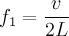and the n'th harmonic is given as fn=nf1.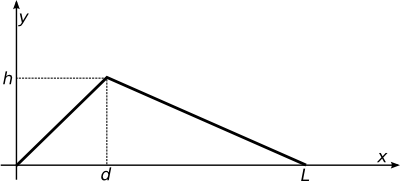Fig. 2: Initial plucked deformation of a guitar string, y(x,0) = f(x).

We consider an initial deformation of the string due to a pluck at a position d along the string, of height h, as shown in Fig. 2. The deformation y(x,0)=f(x) can be expressed as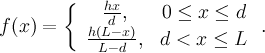We can solve for the spectrum An of excited spatial harmonics as a function of the pluck position d using the orthogonality of the sin(nπx/L) solutions, finding that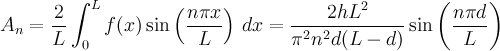We note that, for a typical acoustic guitar the playable length of the string is approximately 26 inches, and the strings are usually plucked or strummed over the body cavity approximately 6 inches from the lower bridge. The spectrum An for this typical case of d/L &asymp 0.23, along with a case in which the string is plucked at d/L=0.5 is plotted in Fig. 3. Note that by symmetry, in the case that d/L=0.5, the amplitudes An=0 for all even n.

### Ideal String with Damping

Of course, any real string includes dissipation due to friction and air resistance, and thus far, our ideal model neglects this contribution. Including dissipative forces, the wave equation (1) is modified to the form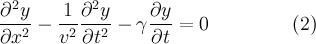where γ is a damping coefficient. For guitar strings, the quality factor of oscillations is very high (they ring a long time after being plucked) such that &gamma is small. A detailed analysis, such as the one provided by De Dayan and Behar, derives a generalized dispersion relation and shows that the presence of damping slightly modifies the harmonics.  However, an earlier experimental investigation by Müller and Wolf showed that for guitar strings this frequency deviation is smaller than a part in 106, and can be neglected.  Rather, the important physical effect introduced by the damping coefficient is a frequency dependent exponential decay of the harmonics. We find that the n'th harmonic is damped by an exponental exp(-t/&taun), where &taun is proportional to 1/(n&gamma). Thus, the introduction of a damping term in Eq. (2) causes a more rapid dissipation of the higher harmonics excited by the pluck.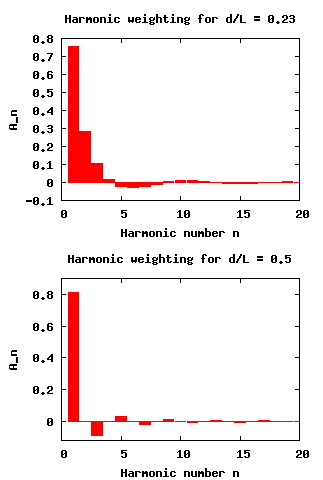Fig. 3: Spectrum An of excited spatial harmonics for d/L=0.23 and 0.5.

## Real String with Stiffness

We have assumed to this point that the string does not stretch as the stress of the pluck is applied, or that the Young's Modulus E of the string material is 0. To incorporate the important effects of stress-strain coupling, we incorporate a stiffness term in the wave equation, such that the governing equation becomes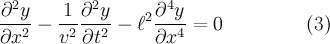where the characteristic length l=(πr4E/4T)1/2 is associated with longitudinal waves that form along the string. This equation is analyzed in great detail by Wolf and Müller, who derive a transcendental equation for the frequencies fn.  They find that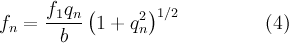where the qn are implicit solutions to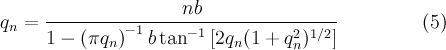where b characterizes the strength of the stiffness term and the string's resulting inharmonicity, and is given by b=πl/L. Evaluating b using parameters common for classical guitar strings,  we find that b≈0.008. For such small b, the second term in the denominator of Eq. (5) is also small, and we can effectively approximate the frequency of the n'th harmonic, rewriting Eq. (3) as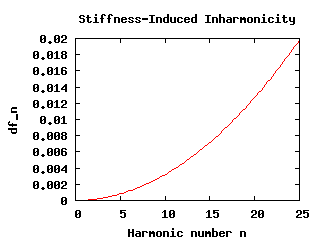Fig. 4: Inharmonicity δfn = (fn-nf1)/nf1 as a function of harmonic number for b=0.008.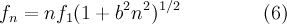In Fig. 4 we plot the fractional deviation of the n'th harmonic from its natural frequency nf1, δfn=(fn-nf1)/nf1, and see that it is not until harmonics n>17 that there begin to be deviations from the natural frequencies on the order of 1%.

## Results and Conclusion

We discretize time and implement the solution to Eq. (3) to create audio files of the sound of a guitar as a function of its pluck position d/L and inharmonicity factor b. The sound waveform of the guitar string is given simply by the temporal part of the solution to the wave equation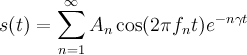where the fn are given by Eq. (6). Representative audio files can be heard by following the hyperlinks in the table to the right.

b d/L
0.00 0.10 10n.wav
0.00 0.23 23n.wav
0.00 0.50 50n.wav
0.03 0.10 10d.wav
0.03 0.23 23d.wav
0.03 0.50 50d.wav
Table 1:Numerically produced guitar string sound files. The C source generating these files is available here.

Each sound file consists of an A major arpeggio followed by an A major chord, with f1=220 Hz corresponding to the A below middle C on a piano. We notice that for d/L=0.1, the sound is much sharper or harsher than for d/L=0.5 due to the strength of the higher harmonics. The examples use a b value higher than is normally found for good guitar strings due to the subtlety of the effect. We find that the presence of dispersion causes a "dulling" of the sound of the string and a "wobbliness" of the pitch, which most listeners describe as a decrease in sound quality.  Also, in the cases for d/L=0.5, the string sounds more like a harp than that of a guitar; harp strings are generally plucked from closer to the center, and therefore contain less higher harmonic content than guitar sounds.

In this project, we have studied a model of a guitar string incorporating the effects of damping and string stiffness analytically. We wrote down the relevant wave equation and produced approximate analytical solutions to the motion of the guitar string. The string sound s(t) was modeled as the temporal solution to the wave equation given a pluck pattern f(x). This, in general, is not all that goes into the sound of a guitar. There is an interaction between the vibration of the string and the body cavity of the guitar, and it is in fact the vibration of the body cavity that produces the sound. The dynamics of such a geometrically complex 3D resonating structure are beyond the reach of analytical methods. In recent years, there has been much numerical work on reproducing the sounds of musical instruments. Effective physical models are instructive not only to instrument designers but to performers as well. One interesting example focusing on guitars models not only the dynamics of the string and resonator, but includes a model of a player's finger interacting with the string. Nevertheless, using analytical techniques we have effectively reproduced the essential elements of the guitar's sound.

© 2007 Jason Pelc. The author grants permission to copy, distribute and display this work in unaltered form, with attribution to the author, for noncommercial purposes only. All other rights, including commercial rights, are reserved to the author.

## References

 Image from the Wikimedia Commons. Accessed 7 December 2007.

 H. G. De Dayan and A. Behar, J. Sound and Vibration 64, 421 (1979).

 H. Müller and D. Wolf, Z. Agnew. Phys. 18, 189 (1964).

 D. Wolf and H. Müller, J. Acoust. Soc. Am. 44, 1093 (1968).

 Data from D'Addario & Co. Inc. Accessed 7 December 2007.

 G. Cuzzucoli and V. Lombardo, Comput. Music J. 23, 52 (1999).